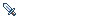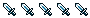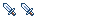sql exec report

Ask for help regarding any technical issue or report any bug or OS independent issues.
mowen
PeonPosts: 11
Joined: 22 May 2009, 19:05

sql exec report

dalstorage.cpp

maybe written like this:

Code: Select all

``````            try
{
std::ostringstream sql;

sql << "Select Id from " << ITEMS_TBL_NAME
<< " Where Id = " << id;

mDb->execSql(sql.str());

sql.str("");

if (mDb->getModifiedRows())
{
sql << "UPDATE " << ITEMS_TBL_NAME
<< " SET name = '" << mDb->escapeSQL(name) << "', "
<< "     description = '" << mDb->escapeSQL(desc) << "', "
<< "     image = '" << image << "', "
<< "     weight = " << weight << ", "
<< "     itemtype = '" << type << "', "
<< "     effect = '" << mDb->escapeSQL(eff) << "', "
<< "     dyestring = '" << dye << "' "
<< " WHERE id = " << id;

mDb->execSql(sql.str());
}
else
{
if (mDb->getModifiedRows() == 0)
{
sql.clear();
sql.str("");
sql << "INSERT INTO " << ITEMS_TBL_NAME
<< "  VALUES ( " << id << ", '" << name << "', '"
<< desc << "', '" << image << "', " << weight << ", '"
<< type << "', '" << eff << "', '" << dye << "' )";
mDb->execSql(sql.str());
}
}

itmCount++;
}
catch (dal::DbSqlQueryExecFailure const &e)
{
utils::Logger::log("execSQL errorÃƒÂ¯Ã‚Â¼Ã…Â¡%s" ,e.what());
}
``````Posts: 4209
Joined: 01 Nov 2007, 18:35
Location: Internet

Re: sql exec report

Can you be more verbose on what you're trying to do?
trapdoor
NovicePosts: 216
Joined: 18 Feb 2007, 13:36

Re: sql exec report

This code was recently changed by me to use prepared statements. This was done to stop sql injection attacks.

--
trapdoor